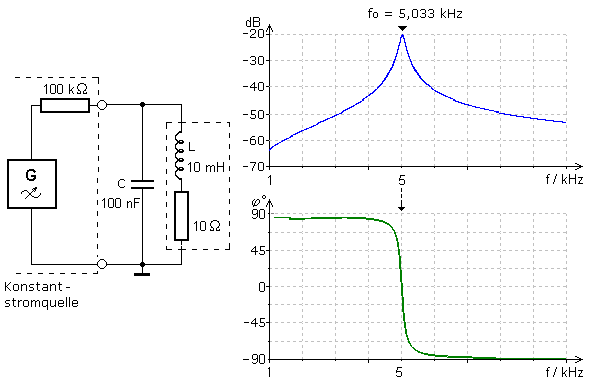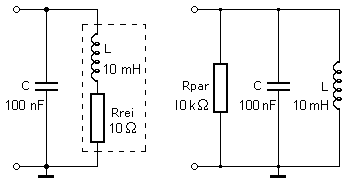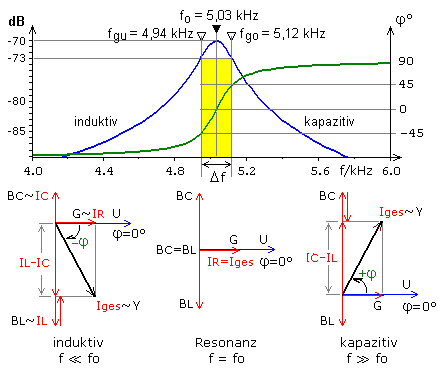# What is the formula for the resonance frequency?

## The parallel resonant circuit

If there are two mutually complementary energy stores and energy converters in the circuit, a single energy supply can lead to dampened resonance oscillations. The process has been clearly illustrated in the chapter Introduction to the resonant circuit. A simple electrical oscillating circuit contains the passive components capacitor and coil. In most cases the capacitor can be seen as the ideal component. The coil with its ohmic wire resistance has a less ideal behavior. A short energy pulse applied once stimulates the oscillating circuit to dampen oscillation with its characteristic natural frequency. Depending on the quality of the components, the oscillation more or less fades away.

If an oscillating circuit is permanently supplied with energy and the signal source has a wide frequency band, then a pronounced signal amplitude can be measured on the oscillating circuit at its resonance frequency. With an oscillating circuit, the resonance frequency of which is adjustable, precisely one desired information can be filtered out of the electromagnetic frequency mixture of many radio and television channels.

The series connection of R, L and C is described in detail in the chapter series resonant circuit. In the parallel resonant circuit, the capacitor and coil form a parallel circuit. Since the ohmic wire resistance of the coils forms a series circuit with the inductive reactance, the value of R must be converted into the equivalent value of a parallel resistance for the exact calculation of the parallel resonant circuit.

The properties of the parallel resonant circuit are examined for the case of external excitation. If a low-resistance voltage source is connected in parallel to the resonant circuit, its internal resistance loads the resonant circuit so much that no resonance can be recognized. The signal source must be connected decoupled via a high-resistance series resistor. Considered together with the series resistor, the signal source behaves as a constant current source. There is almost no load on the resonant circuit and the energy losses in the resonant circuit can only be ascribed to the ohmic resistance of the coil. The circuit simulation provides the amplitude and phase frequency response of the parallel resonant circuit shown in the picture.If the generator voltage is constant, the frequency is continuously changed. As the frequency increases, the voltage on the resonant circuit increases, reaches its maximum at the characteristic resonance frequency fo and then decreases again. In relation to the input signal, the phase shift of the signal on the resonant circuit runs through the range from φ = + 90 ° to φ = −90 ° with φ = 0 ° at the resonance frequency. Viewed over a large frequency range, the curve is not symmetrical on both sides of the resonance frequency. Often only the more important area around the maximum is recorded, where the curve shape appears symmetrical.The circuit shows the real parallel resonant circuit as a mixer circuit. The series connection of inductance and loss resistance is converted into an equivalent parallel connection for the resonance frequency. The formulas are simplified when the value of the ohmic coil resistance is very small compared to the reactance at resonance. This corresponds to a coil quality that is greater than 10. The result is a parallel connection consisting of two reactances and one active resistance, which can be easily described and calculated using vector diagrams.

When determining the phase of a parallel connection, the voltage that is the same across all components is the reference value. Current and voltage at the ohmic resistor do not show any phase shift. In the capacitor branch, the phase position between current and voltage is + 90 °. In the coil branch, the phase position between current and voltage is −90 °. The branch currents are directly proportional to the conductance values ​​with which the vector diagram is created.

For low frequencies, the respective inductive susceptance determines the behavior of the circuit. At high frequencies, the respective capacitive susceptance is decisive. Only at the resonance frequency fo are both susceptibility values ​​equal. Because of their opposite phase relationship, they cancel each other out in their effect. In the case of resonance, the parallel resonant circuit then has the properties of an ohmic resistance and the phase angle between current and voltage is φ = 0 °.In the case of an ideal capacitance and inductance, the resonance frequency and thus Thomson's oscillation equation are derived by equating both reactances or conductance values. In the transmission curve shown above, two further frequencies are shown for the phase angle φ = ± 45 °. As with high-pass and low-pass circuits, limit frequencies can also be defined for the resonant circuit. The parallel resonant circuit behaves more inductively below the resonance frequency and more capacitively above it. At the cut-off frequencies, the amplitude of the resonance curve at −3 dB is still 70.7% of its maximum value. The difference between the upper and lower limit frequency is called the bandwidth.### The bandwidth

In general, the bandwidth in a continuous frequency spectrum is the difference between two frequencies. In the case of resonant circuits and electronic filters, the absolute bandwidth Δf is the range between the two cut-off frequencies. This area is marked yellow in the diagram of the amplitude-frequency response. At the corner frequencies, the amplitude is still 70.7% of its maximum value, which means it has dropped to −3 dB. Absolute bandwidth: B = Δf = fgo - fgu

With given C and L values, the bandwidth of the resonant circuit is influenced by the ohmic parallel resistance. In the vector diagram you can see that with smaller master values ​​G the phase angles φ of −90 ° ... 0 ° ... + 90 ° are passed through much faster. A small conductance, equivalent to a large value of the parallel resistance, has little load on the resonant circuit and the resonance curve is narrower and steeper. In a resonant circuit with ideal behavior, there is no parallel resistance, its value would be infinitely large and the equivalent wire resistance of the coil would tend towards zero. The bandwidth would then also tend towards zero and there would only be an amplitude at the resonance frequency. This case is practically impossible because there are no absolutely ideal components.

### The relative bandwidth

If the absolute bandwidth is much smaller than the resonance frequency, a relative bandwidth Brel can be specified. It is the ratio of the absolute bandwidth B = Δf related to the resonance frequency f0. The result is an unnamed numerical value, which is often given as a percentage. Relative bandwidth: B.rel = Δf / f0

### The quality of the resonant circuit

The longer the energy oscillates in an oscillating circuit that has been triggered or the less energy has to be supplied to maintain a constant oscillation amplitude, the more ideal its behavior. The energy losses are proportional to the ohmic resistance or conductance. The loss factor of a real coil is calculated as the quotient of the ESR (series equivalent resistance) and reactance. The ohmic wire resistance that can be determined with the ohmmeter corresponds to the ESR. No ESR can be measured with a real capacitor. As is currently customary, the determination of the loss factor is based on the series equivalent circuit of the real capacitor. The quality is the reciprocal of the loss factor. The smaller the ESR, the more ideally the component behaves. The ESR values ​​can be converted into their parallel values. In the real parallel resonant circuit, the two reactances and ohmic resistors are connected in parallel. It can be seen that the ohmic wire resistance of the coil is primarily responsible for the energy loss in the form of heat. With its lower quality, the coil consequently determines the no-load quality of the parallel resonant circuit.The no-load quality of a real parallel resonant circuit changes by adding a parallel resistor, by the internal resistance of the exciting signal source and by every additional load connected. Each of these assemblies determines the operational quality of the oscillating circuit, which is always lower than the no-load quality. The absolute bandwidth is also influenced and increases with increasing load. The no-load and the operational quality can be determined from the ratio of the resonance frequency to the −3 dB bandwidth: Q = f0 / Δf

If an oscillating circuit is excited to its resonance frequency by a short energy pulse, the quality can be determined from the decaying oscillation sequence with the oscilloscope. The method delivers useful results up to around 10 MHz. The number of oscillations within which the initial amplitude has decreased by half is counted. The quality is obtained when this value is multiplied by a factor of 5. The picture shows the decaying oscillation for the parallel resonant circuit with the specified dimensioning. The quality values ​​determined in different ways agree quite well. The quality of the coil is the most accurate value because the measurement errors are smaller than the reading errors.### The resonance resistance

In the case of resonance, the values ​​of both reactances are the same, the phase relationships between voltage and current are opposite and cancel each other out. Seen from the outside, the resonant circuit behaves like an ohmic resistor at fo. Without damping by connected assemblies, the resonance resistance Zo is determined by the loss resistance of the coil. In the case of the parallel resonant circuit, this is the converted equivalent parallel resistance.The resonant circuit with the component values ​​given above has its resonance frequency at 5 kHz. The ohmic resistance effective to the outside was calculated to be 10 kΩ and is also obtained using the formula for Zo. This value can be determined in the laboratory by measuring the voltage and current at resonance. The resonance frequency seems to be independent of the coil quality or a corresponding damping resistance. In practice, this applies to high-quality coils and low-attenuated narrow-band parallel resonant circuits. However, the exact calculation shows a small dependence of the resonance frequency on the coil resistance. If the bandwidth of a parallel resonant circuit is increased by adding an ohmic resistor, the resonance frequency becomes somewhat smaller.

### The resonance frequency of the real parallel resonant circuitWith a parallel resonant circuit consisting of C = 100 nF and L = 10 mH, the coil quality was impaired by resistors connected in series. The table shows a slight shift in the resonance frequency to lower values. Since a resonant circuit with an operating quality of less than 10 is practically not sensible, the frequency drift remains negligibly small.

Parallel resonant circuits are often used in the tuner as a tuning circuit for selecting a station, as a working resistor in the resonance amplifier, in oscillators and as a blocking circuit for filtering out narrowly limited frequency ranges. The following is a summary of the most important properties of a parallel resonant circuit:

In the case of resonance, a parallel resonant circuit behaves like an ohmic resistor.
The impedance of the parallel circuit has its greatest value at resonance.
At resonance, the total current flowing into the circuit is smallest.
Due to the resonance increase, the reactive currents in the circuit are maximum and greater than the total current that can be measured outside.
The operational quality is always lower than the no-load quality.
The bandwidth is inversely proportional to the circular quality.
The resonance frequency becomes somewhat lower with increasing circular damping.

### Derivation of the limit frequency equations

The characteristic frequencies of an oscillating circuit are its resonance frequency and the two limit frequencies with which the bandwidth can be determined. They can be determined quite simply experimentally and can also be derived mathematically. By definition, the phase angle at the limit frequencies has the value φ = ± 45 °, with the sign indicating the more inductive or capacitive character of the circuit. The phase angle of | 45 ° | is given when the susceptibility value, in the case of the resonant circuit the difference between the susceptance values ​​for coil and capacitor, is equal to the effective conductance.The bandwidth at resonance depends on the quality of the resonant circuit and is defined as the difference between the upper and lower limit frequency. Without additional damping, the circular quality of the parallel resonant circuit is only determined by the quality of the coil, since the capacitor can be regarded as an ideal component by comparison.In the case of the parallel resonant circuit, the cut-off frequencies are almost symmetrical to the resonance frequency. This statement also applies to the series resonant circuit. The resonance frequency is the center frequency, which is calculated from the geometric mean of the two limit frequencies: fo = √ (fgu · fgo)